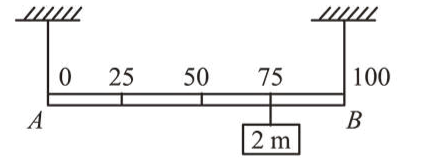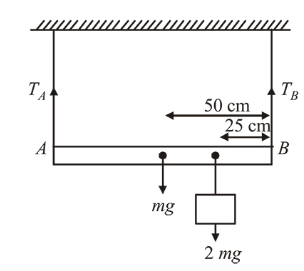# Shown in the figure is rigid and uniform one meter long rod $A B$ held in horizontal positionQuestion:Shown in the figure is rigid and uniform one meter long rod $A B$ held in horizontal position by two strings tied to its ends and attached to the ceiling. The rod is of mass ' $m$ ' and has another weight of mass $2 \mathrm{~m}$ hung at a distance of 75 $\mathrm{cm}$ from $A$. The tension in the string at $A$ is :

1. $0.5 \mathrm{mg}$

2. $2 \mathrm{mg}$

3. $0.75 \mathrm{mg}$

4. $1 \mathrm{mg}$

Correct Option: , 4

Solution:

(4) Net torque, $\tau_{\text {net }}$ about $B$ is zero at equilibrium

$\therefore T_{A} \times 100-m g \times 50-2 m g \times 25=0$

$\Rightarrow T_{A} \times 100=100 \mathrm{mg}$

$\Rightarrow T_{A}=1 \mathrm{mg}($ Tension in the string at $A)$